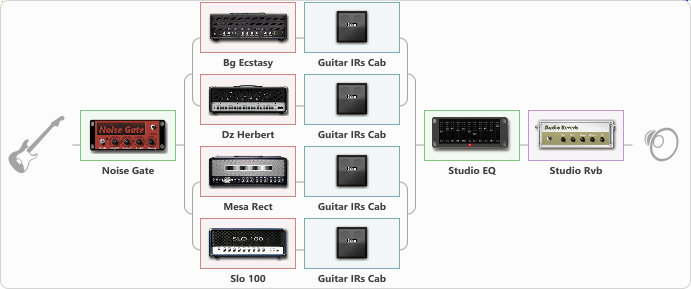# Big Ben Shout

Discussion in 'ToneLib-GFX presets' started by zainal talib, Jan 8, 2021.

1. Big Ben Shout
Preset name: Big Ben

4 types of Hi Gain Amps Sim to 4 cabinets configurations , Experimental try out , Good Luck.

Regards Bdin Hamer

Effects chain:Effect: "Noise Gate" (Dynamics / Filter), active - "yes"
{

"Mode" = Manual
"Depth" = 50
"Threshold" = 50
"Attack" = 0
"Hold" = 5
"Decay" = 250
}

Effect: "Splitter" (Dynamics / Filter), active - "yes"
{

"A-Bypass" = Off
"A-Pan" = 76
"A-Level" = 64
"B-Bypass" = Off
"B-Pan" = -100
"B-Level" = 72
'A' branch:
{

Effect: "Splitter" (Dynamics / Filter), active - "yes"
{

"A-Bypass" = Off
"A-Pan" = 93
"A-Level" = 50
"B-Bypass" = Off
"B-Pan" = 100
"B-Level" = 47
'A' branch:
{

Effect: "Bg Ecstasy" (Amp simulators), active - "yes"
{

"Gain" = 49
"Bass" = 80
"Middle" = 50
"Treble" = 80
"Presence" = 42
"Master" = 61
"Level (dB)" = -2
}
Effect: "Guitar IRs Cab" (Cabinets), active - "yes"
{

"Model" = Engl Pro (4x12")
"Mic Position" = Center
"Mic Distance" = Middle
"Low Cut (Hz)" = 60
"Hi Cut (kHz)" = 20.0
"Mix" = 83
"Level (dB)" = 0
}
}
'B' branch:
{

Effect: "Dz Herbert" (Amp simulators), active - "yes"
{

"Gain" = 50
"Bass" = 50
"Middle" = 50
"Treble" = 50
"Presence" = 50
"Master" = 50
"Level (dB)" = 0
}
Effect: "Guitar IRs Cab" (Cabinets), active - "yes"
{

"Model" = Mesa Rectifier (4x12")
"Mic Position" = Middle
"Mic Distance" = Near
"Low Cut (Hz)" = 60
"Hi Cut (kHz)" = 20.0
"Mix" = 60
"Level (dB)" = 0
}
}
}
}
'B' branch:
{

Effect: "Splitter" (Dynamics / Filter), active - "yes"
{

"A-Bypass" = Off
"A-Pan" = -100
"A-Level" = 46
"B-Bypass" = Off
"B-Pan" = -100
"B-Level" = 48
'A' branch:
{

Effect: "Mesa Rect" (Amp simulators), active - "yes"
{

"Gain" = 50
"Bass" = 50
"Middle" = 50
"Treble" = 50
"Presence" = 50
"Master" = 45
"Level (dB)" = 0
}
Effect: "Guitar IRs Cab" (Cabinets), active - "yes"
{

"Model" = Fender Deluxe Rev (1x12")
"Mic Position" = Middle
"Mic Distance" = Near
"Low Cut (Hz)" = 60
"Hi Cut (kHz)" = 20.0
"Mix" = 63
"Level (dB)" = 0
}
}
'B' branch:
{

Effect: "Slo 100" (Amp simulators), active - "yes"
{

"Gain" = 50
"Bass" = 50
"Middle" = 50
"Treble" = 50
"Presence" = 50
"Master" = 50
"Level (dB)" = 0
}
Effect: "Guitar IRs Cab" (Cabinets), active - "yes"
{

"Model" = Bogner Ecstasy (4x12")
"Mic Position" = Edge
"Mic Distance" = Near
"Low Cut (Hz)" = 60
"Hi Cut (kHz)" = 20.0
"Mix" = 81
"Level (dB)" = 0
}
}
}
}
}

Effect: "Studio EQ" (Dynamics / Filter), active - "yes"
{

"31 Hz" = 0
"62 Hz" = 0
"125 Hz" = -1
"250 Hz" = -3
"500 Hz" = -7
"1 kHz" = -4
"2 kHz" = 2
"4 kHz" = 1
"8 kHz" = 0
"16 kHz" = 0
"Level (dB)" = 4
}

Effect: "Studio Rvb" (Reverberation), active - "yes"
{

"Time" = 7.4
"PreDelay" = 32
"LoDamp" = 0
"HiDamp" = 12
"Mix" = 38
}

Note: You will need to download and install the ToneLib-GFX software to use the preset.

Last edited: Jan 12, 2021